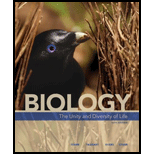Chapter 44, Problem 15SQ### Biology: The Unity and Diversity o...

15th Edition
Cecie Starr + 3 others
ISBN: 9781337408332

#### Solutions

Chapter
Section### Biology: The Unity and Diversity o...

15th Edition
Cecie Starr + 3 others
ISBN: 9781337408332
Textbook Problem
85 views

# Match each term with its most suitable description. _____ carrying capacity a. maximum rate or increase per individual under ideal conditions _____ exponential growth b. population growth plots out as an S-shaped curve _____ biotic potential c. maximum number or individuals sustainable by the resources in a given environment _____ limiting factor d. population growth plots out as a J-shaped curve _____ logistic growth e. essential resource that restricts population growth when scarce

Summary Introduction

To match: The terms with its suitable descriptions.

Explanation

Carrying capacity: c. maximum number of individuals sustainable by the resources in a given environment.

The maximum number of individuals in a population those can withstand in the environment indefinitely by the resources is called carrying capacity. Thus, the population growth attains logistic growth if the population reaches the carrying capacity.

Exponential growth: d. population growth plots out as a J-shaped curve.

The population growth rate becomes more rapid in proportion to the growing total size of population. If the population grows abruptly, it attains the peak value forming a j-shaped curve.

Biotic potential: a. maximum rate of increase per individual under ideal conditions.

Biotic potential is the theoretical rate at which the population grows if the resources are unlimited, and there were no predators and pathogens...

### Still sussing out bartleby?

Check out a sample textbook solution.

See a sample solution

#### The Solution to Your Study Problems

Bartleby provides explanations to thousands of textbook problems written by our experts, many with advanced degrees!

Get Started